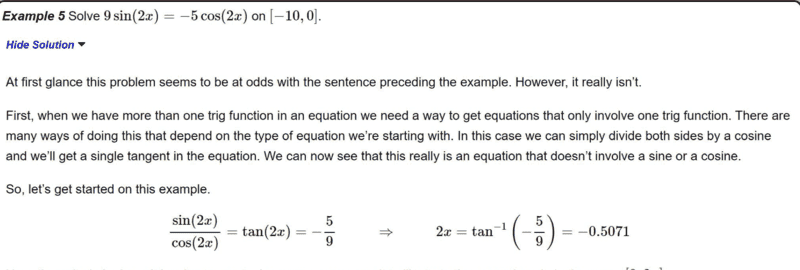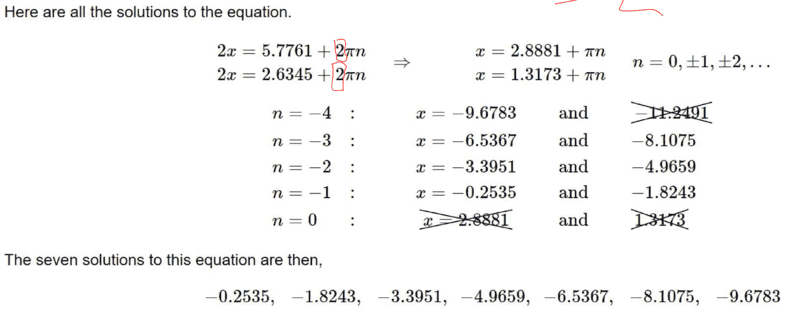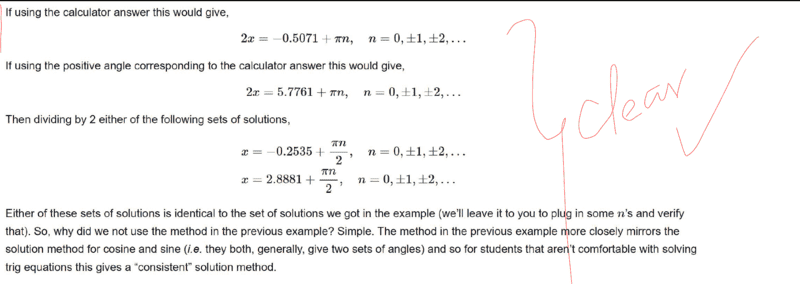# Solve the given trigonometry equation

• chwala

#### chwala

Gold Member
Homework Statement
see attached
Relevant Equations
Trigonometry
This is the problem. The question is simple i just need some clarification as indicated on the part highlighted below in red.Now from my understanding tangent repeats on a cycle of ##π## radians...why do we have 2 the part circled in red below i.e ##2##? This is the part that i need clarity. Why consider cycles for sine and cosine here?
It's straightforward and less stressful to just use ##π##radians in my opinion.The other form given below is the one that i know and am very much conversant with;Last edited:
We know that ##\tan^{-1}####\left[-\dfrac{5}{9}\right]=-0.5071##.

Tangent is negative in the second and fourth quadrant respectively. This will realize the given values: ##-0.5071+π## and ##2π-0.5071## respectively... giving us the desired ##2.6345## and ##5.7761##
i.e
##2x=-0.5071+nπ⇒x=-0.25355+\dfrac{1}{2}nπ##

##2x=2.6345+nπ⇒x=1.31725+\dfrac{1}{2}nπ##

##2x=5.7761+nπ⇒x=2.88805+\dfrac{1}{2}nπ##

where ##n=0, ±1, ±2...## and given that the solution lies on ##-10≤x≤0## then we shall get the answers as shown on the text.

Cheers.

Last edited: